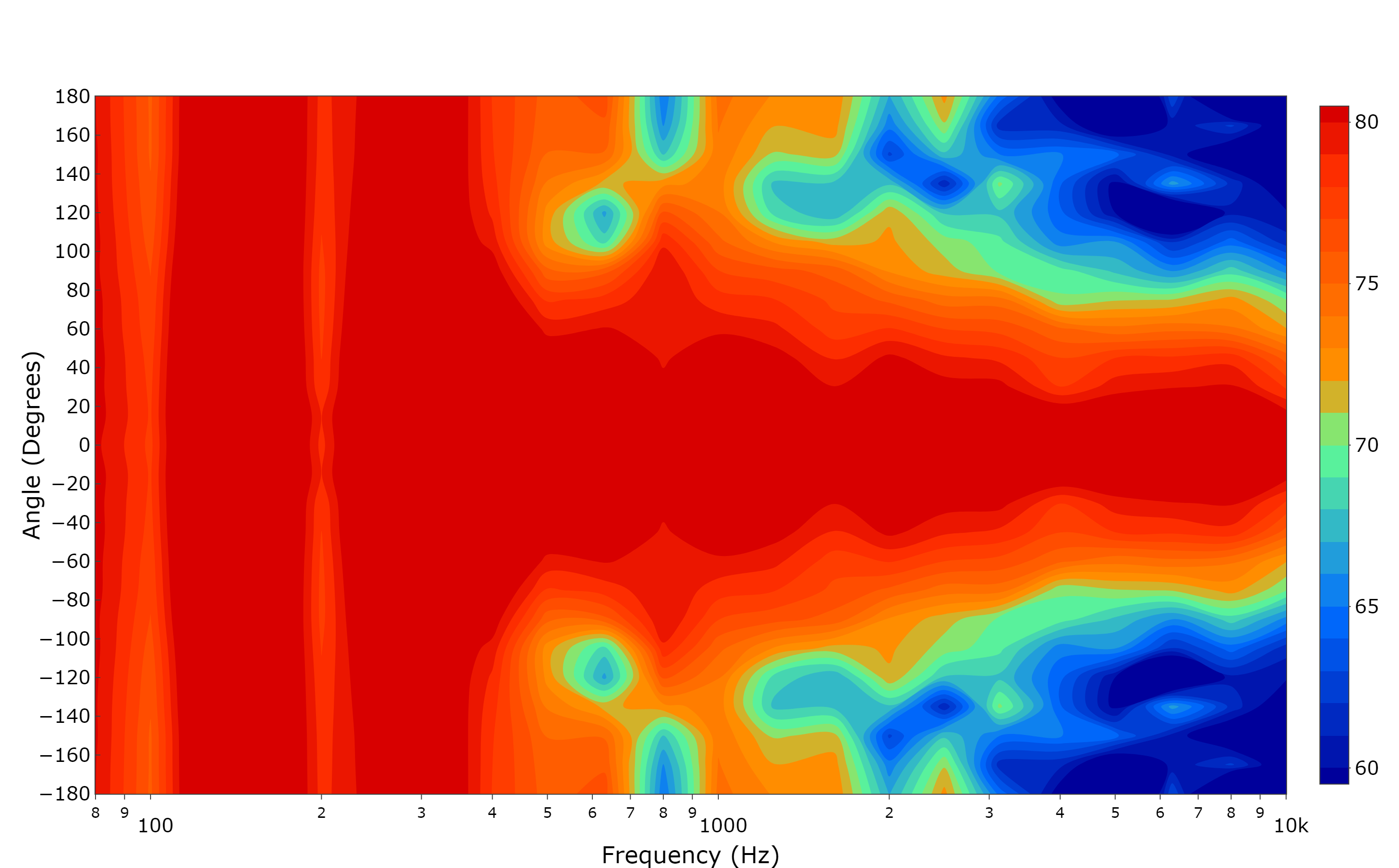# Loudspeaker Directivity PlotsA loudspeaker directivity plot shows frequency response as a function of directivity, allowing you to see off-axis response in comparison to on-axis response. This requires a large number of measurements at different positions, creating quite a large data set.

In this example our data set consists of one column of frequency information (x), one column of measurement angles (y), and a matrix of SPL measurements (z).

Due to the way that data frames are constructed within R (data frames are used to store data in all of the examples given) they are required to have the same number of elements for all columns/rows. This is unlikely to be the case with this data set as, for example, you will have measured at more angles than there are frequency points. To solve this problem we can read data from an Excel file, which will automatically fill in any spaces in our data set. Reading from an Excel file has been avoided in this guide up until now as it generally considered to be more efficient to read from a csv file.

We will need to install the package readxl and use the function read_excel to import our data.

```# check.packages function: install and load multiple R packages.
# Check to see if packages are installed. Install them if they are not, then load them into the R session.
check.packages <- function(pkg){
new.pkg <- pkg[!(pkg %in% installed.packages()[, "Package"])]
if (length(new.pkg))
install.packages(new.pkg, dependencies = TRUE)
sapply(pkg, require, character.only = TRUE)
}

check.packages(packages)
devtools::install_github("ropensci/plotly")

library(tuneR)
library(plotly)

myFile <- file.choose()

Now we need to build a matrix from all of the Z (SPL) data within our spreadsheet. To do this first check how many rows and columns there are:

```#Check the number of rows/columns
nrow(mydata)
ncol(mydata)```

Then we’ll select only the Z data and build the matrix:

```#Extract only Z data and build a matrix
zdata <- mydata[1:22,c(3:27)]
zdata <- data.matrix(zdata, rownames.force = NA)```

Now we are ready to create our plot. We will use type=”contour”.

```p <- plot_ly(x = mydata[],width=1450,height=900)%>%
y = mydata[],
z = zdata,
autocolorscale = FALSE,
autocontour = FALSE,
connectgaps = TRUE,
colorscale = list(
c(0,0.25,0.5,0.6,0.9,1),
c(rgb(0, 0, 155,maxColorValue = 255), rgb(0, 108, 255,maxColorValue = 255),rgb(98, 255, 146,maxColorValue = 255),rgb(255, 147, 0,maxColorValue = 255),rgb(255, 47, 0,maxColorValue = 255),rgb(216, 0, 0,maxColorValue = 255))
),
contours = list(
coloring = "fill",
end = 80,
showlines = FALSE,
size = 1,
start = 60
),
line = list(
dash = "solid",
width = 0.5
),
ncontours = 17,
opacity = 1,
reversescale = FALSE,
showscale = TRUE,
transpose = TRUE,
type = "contour",
xtype = "array",
ytype = "array",
zauto = FALSE,
zmax = 90,
zmin = 50
)%>%```

Unfortunately there is a known bug with setting the colorscale, and so the colours must be set manually. You can find a list of all the default colorscale values here.

The layout section of the script can be very similar to an FFT/Octave band plot shown in the first example.

```layout(
autosize = FALSE,
font = list(size = 20, color="rgb(0, 0, 0)"),
hovermode = "closest",
legend = list(
x = 0.18,
y = 0.819,
borderwidth = 1,
traceorder = "normal"
),
margin = list(
t = 100,
),
paper_bgcolor = "rgba(0, 0, 0, 0)",
plot_bgcolor = "rgba(0, 0, 0, 0)",
showlegend = FALSE,
xaxis = list(
autorange = TRUE,
gridcolor = "rgba(127, 127, 127, 0.5)",
gridwidth = 1,
mirror = TRUE,
range = c(1.3, 4.3),
showline = TRUE,
side = "bottom",
title = "Frequency (Hz)",
type = "log"
),
yaxis = list(
autorange = FALSE,
gridcolor = "rgba(127, 127, 127,0.5)",
gridwidth = 1,
mirror = TRUE,
nticks = 30,
range = c(-180, 180),
showline = TRUE,
side = "left",
ticks = "inside",
title = "Angle (Degrees)",
type = "linear"
)
)```